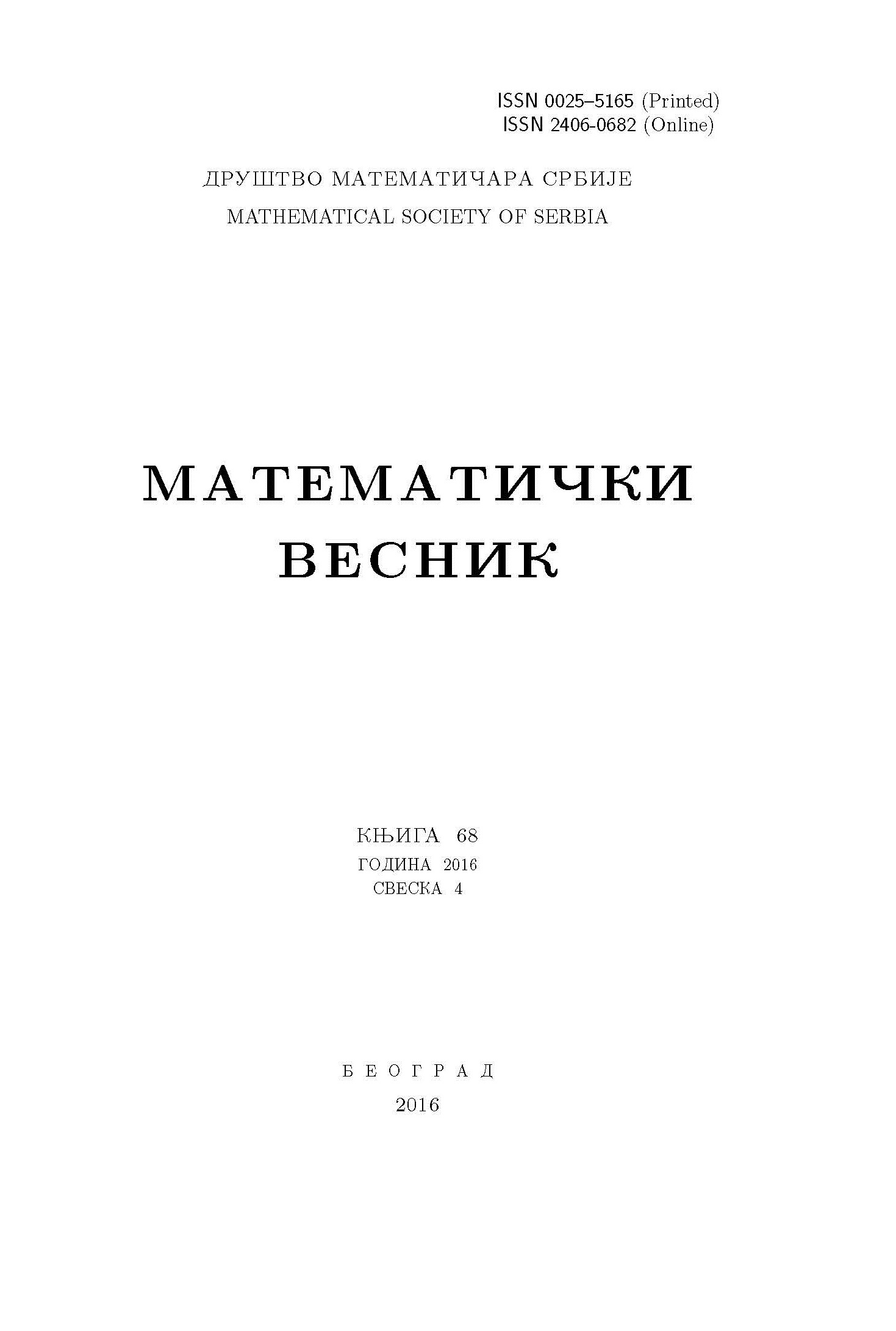﻿ Matematički Vesnik ﻿
 MATEMATIČKI VESNIK МАТЕМАТИЧКИ ВЕСНИКSOME REMARKS ON MONOTONICALLY STAR COUNTABLE SPACES Y.-K. Song, W.-F. Xuan AbstractA topological space $X$ is monotonically star countable if for every open cover $\mathcal U$ of $X$ we can assign a subspace $s(\mathcal U)\subseteq X$, called the kernel, such that $s(\mathcal U)$ is a countable subset of $X$, and $st(s(\mathcal U),\mathcal U)=X$, and if $\mathcal V$ refines $\mathcal U$, then $s(\mathcal U)\subseteq s(\mathcal V)$, where $st(s(\mathcal U),\mathcal U)=\bigcup\{U\in\mathcal U:U\cap s(\mathcal U)\neq\emptyset\}.$ In this paper we study the relation between monotonically star countable spaces and related spaces, and we also study topological properties of monotonically star countable spaces.Keywords: Star finite; monotonically star finite; star countable; monotonically star countable; star Lindel{ö}f; monotonically star Lindel{ö}f. MSC: 54D20, 54D30, 54D40 Pages:  1$-$7

﻿